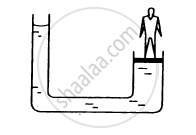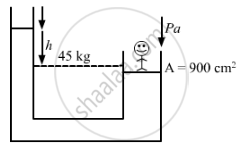Department of Pre-University Education, KarnatakaPUC Karnataka Science Class 11
Advertisement Remove all ads

# The Area of Cross Section of the Wider Tube Shown in Figure is 900 Cm2. If the Boy Standing on the Piston Weighs 45 Kg, Find the Difference in the Levels of Water in the Two Tubes. - Physics

Answer in Brief

The area of cross section of the wider tube shown in figure is 900 cm2. If the boy standing on the piston weighs 45 kg, find the difference in the levels of water in the two tubes.Advertisement Remove all ads

#### Solution

Given:
Area of the wider tube, A = 900 cm2
Weight of the boy, m = 45 kg
Density of water, ρ = 10kgm−3
Let h be the difference in the levels of water in the tubes and pa be the atmospheric pressure.As per the figure, we have:

Pa + hρg = Pa +(mg)/A

=> hρg =(mg)/A

=> h = m/(ρA)

=> h = m/(1000×A)

=45/(1000xx900xx10^(-4))=1/2m = 50cm

Is there an error in this question or solution?
Advertisement Remove all ads

#### APPEARS IN

HC Verma Class 11, 12 Concepts of Physics 1
Chapter 13 Fluid Mechanics
Q 3 | Page 273
Advertisement Remove all ads
Advertisement Remove all ads
Share
Notifications

View all notifications

Forgot password?